## Friday, March 29, 2013

### HP 39gii Programming Tutorial - Part 5: Pixels and Graphics

Welcome everyone to Part 5 of my HP 39gii programming tutorial.

We are going to work with some graphic commands with the HP 39gii.

The Screen

When it comes to graphics, the HP 39gii has two systems: points and pixels.

Points refers to Cartesian points. Points work just as you would expect: x increases as you go right, y increases as you go up. The boundary of the screen are controlled by the value of Xmin, Xmax, Ymin, and Ymax.

Pixels refer to the LCD terminal screen. Unless you are working with the Plot screen, you are most likely going to work with the LCD terminal screen. In the pixel system, each coordinate is fixed. The 39gii screen is 255 pixels by 126 pixels. In the pixel system, the upper left hand coordinate is (0,0), and the bottom right hand corner is (254,125). The x coordinate increases as you go to the right, but the y coordinate increases as you go down. A row of (about) 14 pixels is reserved for the soft key menus (F1 - F6). In practice you would deal a screen of size 255 × 111.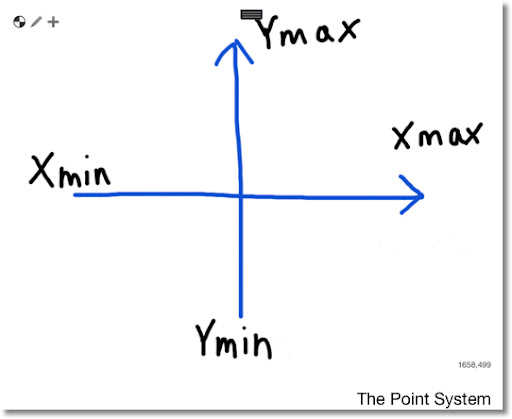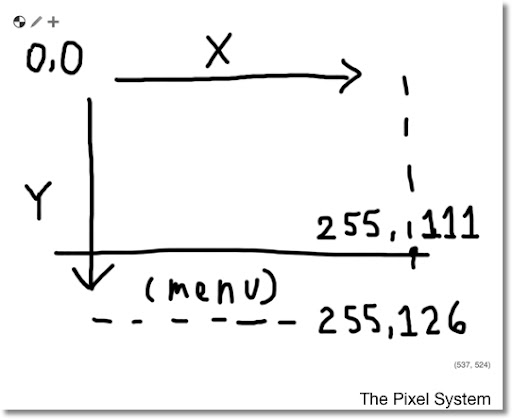We will work with the Pixel system in this part of the tutorial.

Common Drawing Commands

In a program, you will want to erase the drawing screen, and at the end, you will need a "hold" command.

Erasing the screen is pretty simple. Whenever you want to erase the LCD screen, type RECT();.

The "hold" command freezes the screen together to show you the picture. Without the "hold" command the calculator just returns to the Home screen. Two "hold" commands are WAIT(0) and FREEZE. I prefer WAIT(0) because the program continues with the press of any key and it works as expected.

Some of the common drawing commands are:

Erase the drawing screen:

RECT();

Key Sequence:
[SHIFT] [Math] [ F1 ] [ 4 ] [ ) ]

To put text anywhere on the screen, starting from pixel (x,y):

TEXTOUT_P(string,x,y);

Key Sequence:
[SHIFT] [Math] [ F1 ] [ 4 ] [ up ] [ENTER]

To draw a line segment from pixel (x1, y1) to (x2, y2):

LINE_P(x1,y1,x2,y2);

Key Sequence:
[SHIFT] [Math] [ F1 ] [ 4 ] [ LN ]

To draw a white box with black border, given opposite corner pixels (x1, y1) and (x2, y2):

RECT_P(x1,y1,x2,y2,0,3);

Key Sequence:
[SHIFT] [Math] [ F1 ] [ 4 ] [ ÷ ]

To draw a circle with center pixel (x,y) and radius of r pixels:

ARC_P(x,y,r);

Key Sequence:
[SHIFT] [Math] [ F1 ] [ 4 ] [ 2 ]

To draw an arc between angle A and B:

ARC_P(x,y,r,A,B);

Key Sequence:
[SHIFT] [Math] [ F1 ] [ 4 ] [ 2 ]

To turn the pixel (x,y) on:

PIXON_P(x,y);

Key Sequence:
[SHIFT] [Math] [ F1 ] [ 4 ] [ ( ]

To turn the pixel (x,y) off:

PIXOFF_P(x,y);

Key Sequence:
[SHIFT] [Math] [ F1 ] [ 4 ] [ x² ]

Colors (Really Grayscale) on the 39gii

The 39gii has four grayscale shades. Each have the following values:
0 = black
1 = dark gray
2 = light gray
3 = white

You can use color in the following commands. The color arguments are always optional.

RECT_P(x1,y1,x2,y2,edge color,fill color);
LINE_P(x1,y1,x2,y2,color);
PIXON_P(x,y,color);
ARC_P(x,y,r,A,B,color);

Fonts with the 39gii

The HP 39gii has two fonts: large and small. They have the following values:
0 (or left out) = default, whatever the system setting is
1 = force small font
2 = force large font

The syntax of TEXTOUT_P with font size is:
TEXTOUT_P(string,x,y,font size);

Let's use these commands in some programs.

The Program RTEXT

RTEXT(desired string, number of places)

RTEXT places a desired string all over the screen at random.

EXPORT RTEXT(str1,times)
LOCAL X,Y,K;
RECT();
RANDSEED(RANDOM);
FOR K FROM 1 TO times DO
X:=INT(RANDOM(1,254));
Y:=INT(RANDOM(1,111));
TEXTOUT_P(str1,X,Y);
END;
WAIT(0);
END;

Example: Sprinkle "39gii" in 75 random places. From the Home screen type RTEXT("39gii",75). You get something like this: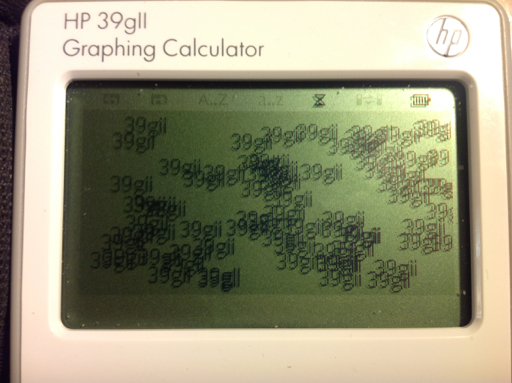The Program BOXLH

The program BOXLH draws a box of length L and height H. The box grows from the center pixel (127, 56). Input: BOXLH(L,H)

EXPORT BOXLH(L,H)
BEGIN
RECT();
RECT_P(127-L/2,56-H/2,127+L/2,56+H/2,0,3);
WAIT(0);
END;

Example:
Draw a box with length of 60 pixels and height of 56 pixels. From the home screen type BOX(60,56).The Program BOX3D

BOX3D takes the box drawing program one step further, by adding some depth to make the box look three-dimensional.

Input: BOX3D(L,H,D,A)
L = length of the box in pixels
H = height of the box in pixels
D = depth of the box in pixels
A = tilt angle in degrees

BOX3D sets the angle mode to Degrees. (HAngle = 0).

EXPORT BOX3D(L,H,D,A)
BEGIN
LOCAL X,Y,Z,T,C,S;
HAngle:=1;
RECT();
X:=127-L/2;
Y:=127+L/2;
Z:=56-H/2;
T:=56+H/2;
RECT_P(X,Z,Y,T,0,3);
C:=D*SIN(A);
S:=D*COS(A);
RECT_P(X+C,Z-S,Y+C,T-S,0,3);
LINE_P(X,Z,X+C,Z-S);
LINE_P(Y,Z,X+C,Z-S);
LINE_P(X,T,X+C,T-S);
LINE_P(Y,T,Y+C,T-S);
WAIT(0);
END;

Note: The order of the drawing commands is important, especially when it comes to the RECT and RECT_P commands.

Example: Length of 45 pixels, height of 27 pixels, depth of 33 pixels, at an angle of 45°
Enter BOX3D(45,27,33,45)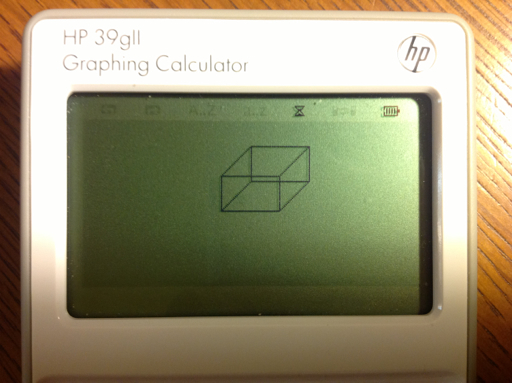A Short Animation: The Program GROWTH

GROWTH is a program of an arc that grows by π/6 (30°) every second until a circle is completed. Radians angle mode (HAngle=1) is set in this program.

EXPORT GROWTH()
BEGIN
RECT();
HAngle:=0;
FOR K FROM 0 TO 2π STEP π/6 DO
ARC_P(127,56,30,0,K);
WAIT(1);
END;
TEXTOUT_P("Done",0,0);
WAIT(0);
END;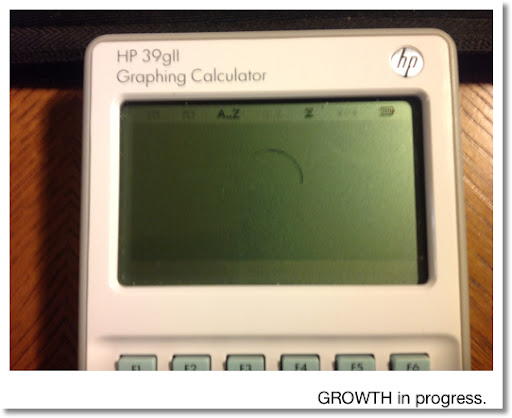CDEMO: Colors and Fonts

CDEMO shows the three colors and the small font. You can cut down on the lines needed by using a clever mathematical equation and a well set up FOR loop.

EXPORT CDEMO()
BEGIN
LOCAL K;
RECT();
FOR K FROM 1 TO 3 DO
RECT_P(100,30*K,200,30*K+25,0,K);
END;
TEXTOUT_P("Dark Gray",1,35,1);
TEXTOUT_P("Light Gray",1,65,1);
TEXTOUT_P("White",1,95,1);
TEXTOUT_P("39gII Colors",50,1,2);
WAIT(0);
END;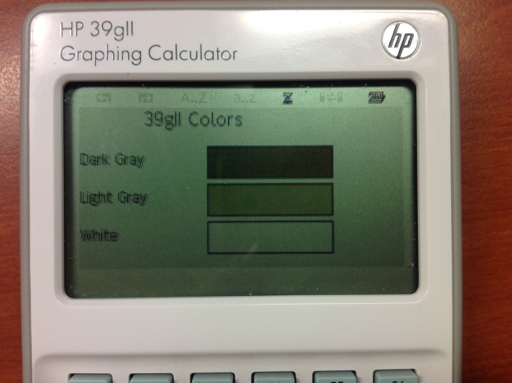Well, I hope these five programs demonstrate what you can do with the graphing commands with the 39gii. As always, I hope encourages you to program and to try things out!

In Part 6, we are going interactive!

Until next time,

Eddie

This blog is property of Edward Shore. 2013

## Saturday, March 23, 2013

### Spring has Sprung: Pocket Ref, TI-30XA, Old HP Manuals, Review and more HP 39gii is coming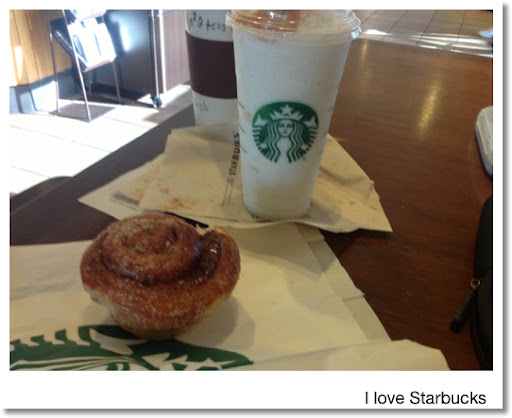Hi everyone! Greetings from the Starbucks in Azusa, CA. Today's blog entry is a hodgepodge. Part 5 of the HP 39gii Programming tutorial - I am planning to have that ready by next week; totally apologize for the long delay.

I am very thankful my family is doing fine and my dad and uncle are both home from the hospital.

Pocket Ref: A Book of Everything?

While at Harbor Freight I found this book: "Pocket Ref 4th Edition" compiled by Thomas J. Glover; picked it up for \$10. This reference edition has practically everything technical: physics, computer, mechanics, construction, automotive equations, electrical engineering equations, table of elements and chemical molecules, weights, densities, and perpetual calendars; just to name a few topics. This book has become a permanent part of things I take everywhere. Hopefully this reference will be used for future blog entries.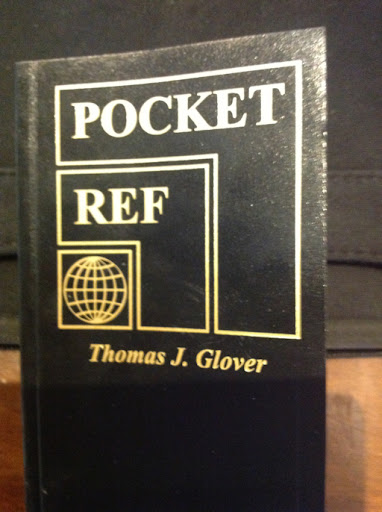The TI-30XA: Then and Now

I finally broke down and got a new version of the TI-30XA by Texas Instruments. I have been resisting it for so long since I preferred the solar version over the battery version. What got me to finally purchase it is the article I saw on www.datamath.org, which details the logarithm bug.

Basically, the calculation goes haywire for:

(1) ln(1+x) when x is really small, and

(2) (1+1/n)^n for really large n.

I tested both calculations for the calculators. I also used the built in calculator in the google.com search engine. If you type in a mathematical calculation in google, it will get you the answer! Google also handles conversions and graphing functions.

Both the TI-30XAs (solar and battery) returned the same answers, so nothing has changed in the algorithm.

(1) ln(1 + x)

x=10^-2
TI-30XA: 0.009950331

x=10^-8
TI-30XA: 0.000000001

(2) (1+1/n)^n

n = 10^2
TI-30XA: 2.70481383

n = 10^8
TI-30XA: 2.7183727

Out of curiosity, the TI-84+ agrees with Google. So this is a cautionary tale with the TI-30XA: be careful with arguments involving 1+x, where x is real small (like powers of 10^-6 or smaller) in any calculations involving logarithms and powers.

On the plus side, the battery TI-30XA retains memory when the ON button is pressed and the numbers are larger and in bold.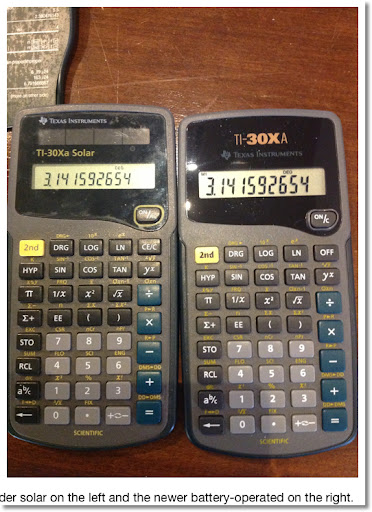Review of the TI-84+ Color Silver Edition Coming Soon

Speaking of Texas Instruments calculators, my TI-84+ C Silver Edition is going to arrive at my doorstep in the coming week. That was my birthday present to myself. By clicking in this sentence, you can read how I initially felt about the TI-84+ CSE. I did read good reviews about it (This sentence is a link to one review, done by Christopher Mitchell (Cemetech). )and will give an impression when I actually have a TI-84+ CSE in my hands.

Old Hewlett Packard Manuals: They have a calculation manual for everything!

Back in the 1970s and 1980s, when pocket calculators were in their beginning stages, Hewlett Packard released detailed calculator manuals for not only their products (HP 35, HP 45, HP 67, etc), they had manuals covering almost every topic conceivable. To name a few: engineering, finance, games, biology, and one even covered astrology. Every now and then I like to study some of these manuals and try to see if I can do it (mostly with today's calculators). It is a really fun way to pass the time.

Several years ago, I ordered the DVD that contains a collection of manuals from The Museum of HP Calculators. You can order the DVD by clicking on this link.. The link will contain another link of the manuals the DVDs contain. I hope to refer to these manuals in future blog entries.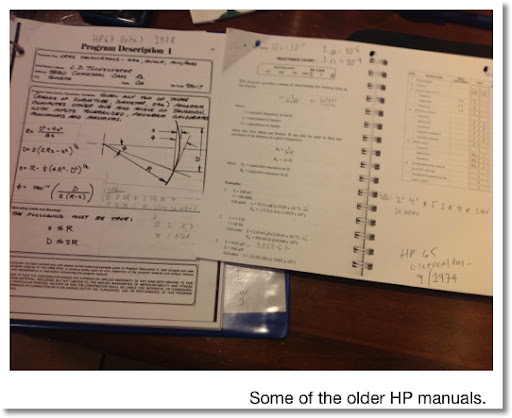So, more HP 39gii Programming Tutorial, a review of the TI-84+ CSE, and more is coming. Thank you as always and take care,

Eddie

This blog is property of Edward Shore. 2013

## Monday, March 18, 2013

### HP 39gii Programming Part 4: REPEAT and WHILE

Welcome to Part 4 of the HP 39gii Programming Tutorial. Here is what is on the menu tonight:

1. REPEAT structure
2. WHILE structure
3. Some Notes
4. The Program EUCLID
5. The Program RSUM1
6. The Program SQFACTOR

This lesson covers the REPEAT and WHILE loops. Each loop repeats a set of instructions indefinitely until a condition is met.

1. The REPEAT Structure

REPEAT
these instructions;
UNTIL this condition is true;

The REPEAT loop executes the set of commands then it tests the condition. Hence in a REPEAT loop, the commands are always executed at least once.

Keystrokes to find the template: In the program editor press [ F6 ] (TMPLT) [ 4 ] [ 4 ]

2. The WHILE Structure

WHILE this condition is true DO
these instructions;
END;

The WHILE loop tests the condition before executing the set of commands. It is possible that the commands in a WHILE loop are never executed.

Keystrokes to find the template: In the program editor press [ F6 ] (TMPLT) [ 4 ] [ 3 ]

3. Some Notes

Loops (FOR, WHILE, REPEAT) can be nested That is, loops can contain other loops. The is demonstrated in the SQFACTOR program, and will be demonstrated in future programs.

PRINT does not need the string command - expressions will evaluate automatically. This will allow to combine strings and mathematical expressions quickly with just the use of the plus sign (+) and the quotation marks (" "). MSGBOX makes everything in its argument a string, so it is best to use the string command. The program listed below demonstrates the difference.

EXPORT TEST814()
BEGIN
MSGBOX("TEST" + 300 + 16);
PRINT(15*20+4² + " IS THE DATE");
END;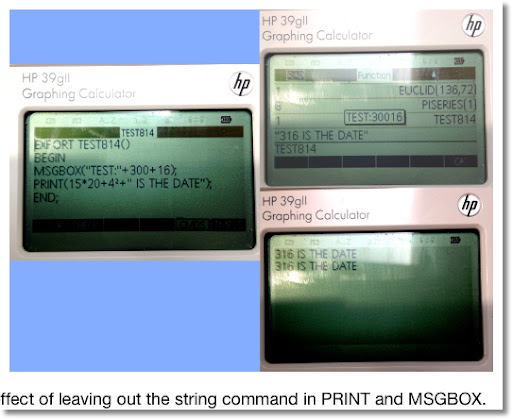4. The Program EUCLID

The goal of EUCLID is to use the Euclidean algorithm to find the greatest common divisor of two integers. The integers can be entered in any order. EUCLID takes the two integers as pass-through arguments.

The program EUCLID
EXPORT EUCLID(X,Y)
BEGIN
LOCAL M,N,R,A;
PRINT();
N:=MAX(X,Y);
M:=MIN(X,Y);
REPEAT
A:=INT(N/M);
R:=N - A*M;
PRINT(A + "*" + M + "+" + R);
WAIT(1);
N:=M;
M:=R;
UNTIL R==0;
RETURN N;
END;

The integer is split into its integer of the quotient, A, and remainder, R, as such:

N = A * M + R

The program repeats until R=0.

Examples:
EUCLID(145,54) → 1
EUCLID(136,72) → 8 (we have a picture of this example)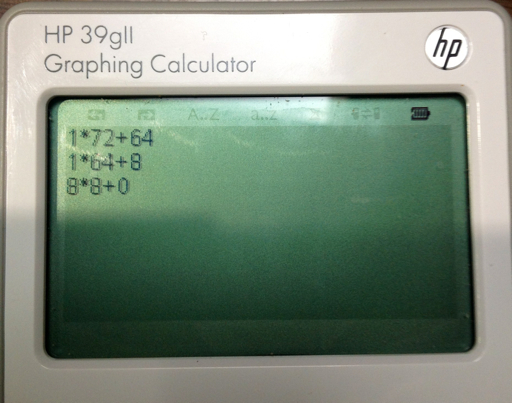5. The Program RSUM1

The program RSUM1 builds a sum of random numbers between 0 and 1 while the total sum is less than 1. RSUM1 takes no arguments.

The Program RSUM1

EXPORT RSUM1()
BEGIN
LOCAL S;
S:=0;
WHILE S<1 DO
S:=S+RANDOM;
END;
RETURN S;
END;

You can find the RANDOM command in the Math-Probability menu ([Math] [Math] [ 4 ]). To generate random numbers between 0 and 1, either RANDOM or RANDOM() is acceptable.

Example sums you may get with RSUM1 are 1.29441419992, 1.28901948188, and 1.57880668903.

6. The program SQFACTOR

The program SQFACTOR simplifies √N where N is an integer (i.e. √180 = 6 √5, √364 = 2 √91). It has one pass-through argument, N.

I am going to use the store arrow (STO>) accessed by the [ F1 ] key in the program editor. The store arrow is a thick dark arrow pointing to the right (▸). You can use the := assignment if you wish.

SQFACTOR(N)
EXPORT SQFACTOR(N)
BEGIN
LOCAL C,K;
1 ▸ C;
2 ▸ K;
WHILE K² < N DO
WHILE FRAC(N/K²) == 0 DO
N/K² ▸ N;
C*K ▸ C;
END;
K+1 ▸ K;
END;
RETURN string(C)+"√"+string(N);
END;

Examples:
SQFACTOR(180) returns "6 √ 5"
SQFACTOR(77) returns "1 √ 77"
SQFACTOR(400) returns "4 √ 25"

OK The last one should be 20. However 4 √25 = 4 × 5 = 20. So this isn't perfect.

Challenge: can this be improved? I will leave this for the readers.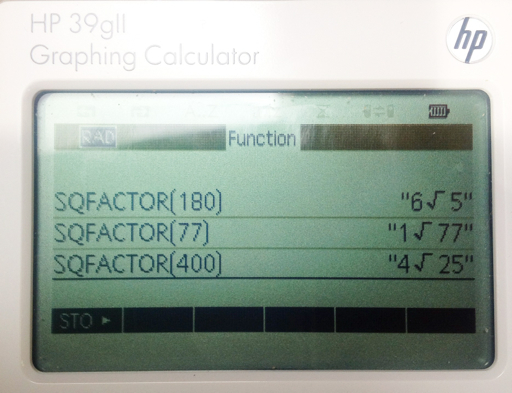That is it for Part 4. Next time, we will cover basic graphics commands. Take care and have fun!

Eddie

This blog is property of Edward Shore. 2013

## Saturday, March 16, 2013

### Decimal/Binary Conversions for the HP 39gii

Decimal and Binary Conversions
Programs created 2/20/2013

Decimal to Binary (number → string)

D2B(number) - execute from Home!

EXPORT D2B(N)
BEGIN
LOCAL str1;
D:=INT(N);
L:=INT(LN(D)/LN(2));
str1:="1";
D:=D-2^L;
FOR K FROM 1 TO L DO
IF 2^(L-K)≤D THEN
str1:=str1+"1";
D:=D-2^(L-K);
ELSE
str1:=str1+"0";
END;
END;
RETURN str1;
END;

Examples:

D2B(52) returns "110100"

D2B(131) returns "10000011"

Binary to Decimal (string → number)

Run this from Home! B2D(string of 1s and 0s). Attempting to run B2D from the program catalog will cause an error.

EXPORT B2D(str1)
BEGIN
D:=dim(str1);
N:=0;
FOR K FROM 0 TO D-1 DO
N:=N+2^K*expr(mid(str1,D-K,1));
END;
RETURN N;
END;

Examples:

B2D("11011") returns 27.

B2D("1010110000") returns 688.

Eddie

This blog is property of Edward Shore. 2013

### HP 39gii Programming Part 3: FOR Loops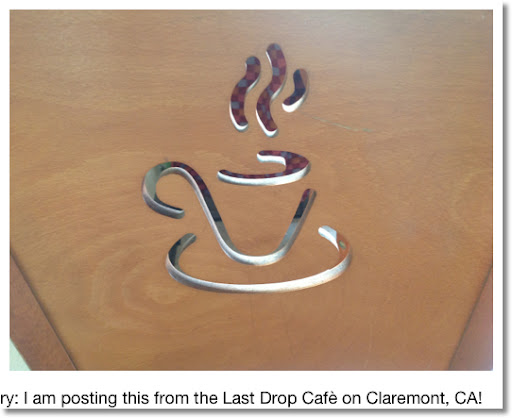This blog entry: I am posting this from the Last Drop Cafè on Claremont, CA - in the college town near the Claremont Colleges. Quite a nice, cozy place. Good coffee too!

-------

Welcome to Part 3!

1. FOR Loops
2. Other Commands Used in this Lesson
3. The program CROOTS
4. The program POLYSYN
5. The program TIMER

1. FOR Loops

The FOR loop is good construct when you want to repeat a set of commands an amount of times. The commands may (or may not) involve a calculation involving a counter variable. For each pass, the value of counter is incremented (or decremented) until counter matches a target number.

An example is:

FOR K FROM 1 TO 4 DO
A:=A+K;
END;

The counter variable in this example is K. For the first pass, K=1. After 1 is added to A, then the loop ends. K increases by 1. For the second pass, K=2. On so on until the fourth pass where K=4. At that point, K is at its target value and the loop ends.

The increment can be any value you want. If you want the increment to be something other than 1, you can specify that by the STEP instruction. For example,

FOR K FROM 1 TO 4 STEP 0.5 DO
A:=A+K;
END;

Here we have specified the step size to be 0.5, which means that with each pass, K increases by 0.5 (from 1 to 1.5 to 2 to 2.5 and so on until K=4).

The FOR loop for the HP 39gii has four general formats.

Basic FOR loop: Increase counter by an increment of 1:

FOR counter FROM start_value TO end_value DO
insert commands here
END;

Keystrokes for this template (in the program editor): [ F6 ] (TMPLT) [ 4 ] [ 1 ]

FOR loop: Increase counter by an increment other than 1:

FOR counter FROM start_value TO end_value STEP increment DO
insert commands here
END;

Keystrokes for this template (in the program editor): [ F6 ] (TMPLT) [ 4 ] [ 2 ]

For loops with where the value of counter decreases, use the following two formats. Please note at the time of this posting, DOWNTO is an undocumented feature.

FOR loop: Decrease counter by a decrement of 1:

FOR counter FROM start_value DOWNTO end_value DO
insert commands here
END;

FOR loop: Decrease counter by a decrement other than 1:

FOR counter FROM start_value DOWNTO end_value STEP decrement DO
insert commands here
END;

I prefer just typing out the FOR-FROM-DOWNTO/TO-STEP-END structure manually.

2. Other Commands Used in this Lesson

The global variable HAngle. This is the master angle setting. Assigning the following values will result as:

1: Degrees Mode
2: Current Mode

How to find HAngle, if you don't want to type it: [Vars] [ F1 ] [ 4 ] [ 2 ]

CONCAT This command puts two lists together as one.

CONCAT(left list, right list)

Example: CONCAT({1,2,3},{5,6,8}) returns {1,2,3,5,6,8}.

Keystrokes: [Math] [ F1 ] [ 7 ] [ 1 ]

EDITLIST

Syntax: EDITLIST(L#);

This puts the calculator in list editing mode. The command edits the list L# where # is a digit 0-9. You can use a localized list name if you wish instead of the global variable L#.

WAIT

Syntax: WAIT(n);

If n=0, the calculator waits indefinitely. Press any key to have the calculator continue. This is great for freezing the screen to display certain results.

If n>0, the calculator waits n seconds before executing the next step. Useful in loops and displaying multiple bits of information. n does not have to be an integer.

Keystrokes: [SHIFT] [Math] [ F1 ] [ 5 ] [Vars]

The three programs CROOTS (Complex Roots), POLYSYN (Synthetic Division), and TIMER will demonstrate these commands.

3. The Program CROOTS

The first program to demonstrate the power of the FOR loop is the program CROOTS. This program finds all the complex roots of a given number. CROOTS finds all the Nth roots of Z and returns the answer in a list.

Input: CROOTS(Z,N)

The program CROOTS:

EXPORT CROOTS(Z,N)
BEGIN
LOCAL L3,K;
HAngle:=0;
L3:={};
FOR K FROM 0 TO N-1 DO
L3:=CONCAT(L3, {N NTHROOT (ABS(Z)) * e^( i * ( ARG(Z) + 2 * K * π )/N)});
END;
RETURN L3;
END;

Examples (answers are rounded to 5 decimal places)

CROOTS(4,2) returns [2, -2 - 2.5335E-12 * i]. In essence, [2, -2]

CROOTS(-1, 3) returns [0.5 + 0.86603*i, -1*, 0.5 - 0.86603*i].

* -1 - 1.26676E-12*i

4. The Program POLYSYN

Description of POLYSYN:

POLYSYN is a program that divides the polynomial

p(x) / (x-R)

by the use of synthetic division. POLYSYN has no pass-through arguments. The program prompts L1, which is a list of coefficients of descending power to the constants. Please include zeroes whenever necessary. The value of R is also prompted.

The result is a list of coefficients, with the last term the remainder

EXPORT POLYSYN()
BEGIN
LOCAL K,S,T;
EDITLIST(L1);
INPUT(R,"P(X)/(X-R)");
L2:=L1;
S:=SIZE(L1);
T:=S-1;
FOR K FROM 1 TO T DO
L2(K+1):=R * L2(K) + L1(K+1);
END;
MSGBOX("LAST TERM=REMAINDER");
RETURN L2;
END;

L1, R, and L2 are permanently updated.

Example 1: (21x^2 + 42x + 144)/(x - 12) = 21x + 294 + 3672/(x-12)

Inputs:
L1 = {21, 42, 144}
R = 12

Output:
L2 = {21, 294, 3672}

Example 2: (x^3 - 5x + 1)/(x + 5) = x^2 - 5x + 20 - 99/(x + 5)

Input:
L1 = {1, 0, -5, 1}
R = -5

Output:
L2 = {1, -5, 20, -99}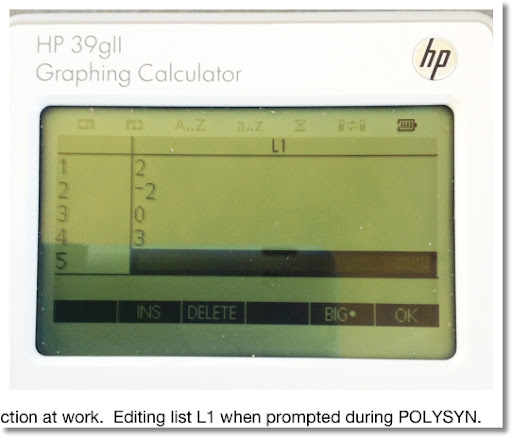5. The program TIMER

TIMER demonstrates a FOR loop with DOWNTO. The program takes one pass-through argument: the number of seconds.

The program TIMER:

EXPORT TIMER(N)
BEGIN
LOCAL K;
FOR K FROM N DOWNTO 1 DO
PRINT(K);
WAIT(1);
END;
END;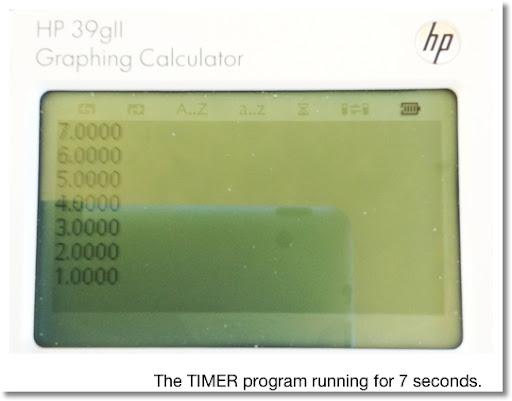In Part 4 of the HP 39gii Series, we will deal with REPEAT And UNTIL.

As always, thank you - I am grateful for your comments and following this blog. Have a great day!

Eddie

This blog is property of Edward Shore. 2013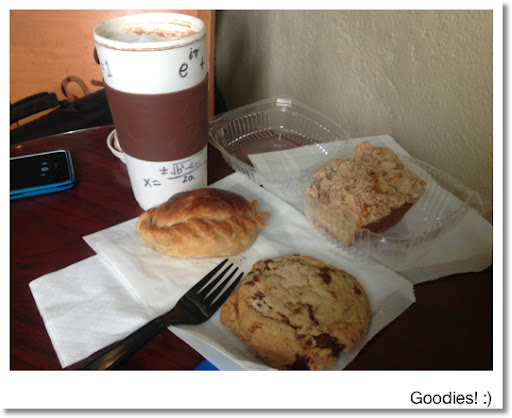## Thursday, March 14, 2013

### Happy π Day! And some Mathematical Art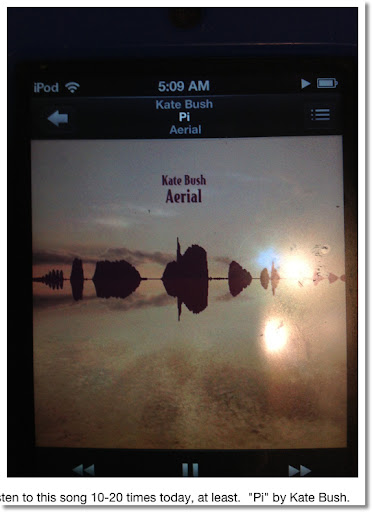Happy π Day!

π = 3.14159 26535 89793 23846 26433 83279 50288...

Who would have known that the ratio of a circle's circumference to its diameter would generate interest though out human history? π never ends, and over 10 trillion digits have been calculate. I have memorized about 15 digits. The digit zero (0) does not appear until the 32nd digit.

Why is π such a "celebrity" number? Find out here:

According to Dr. James Grime, we can calculate the size of the observable universe using π with only 39 digits.

You can use the (very slow converging series) to get π:

π = 4 × (1 - 1/3 + 1/5 - 1/7 + 1/9 - 1/11 + 1/13 - 1/15 + 1/17...)

There are many songs about π. My favorite is "Pi" by Kate Bush (off the album "Aerial").

Tonight I plan to join desmos.com to participate in a π Day discussion.

To celebrate my birthday and π Day, I am going to share some mathematical artwork, which was created using the TI nSpire CX CAS iPad App.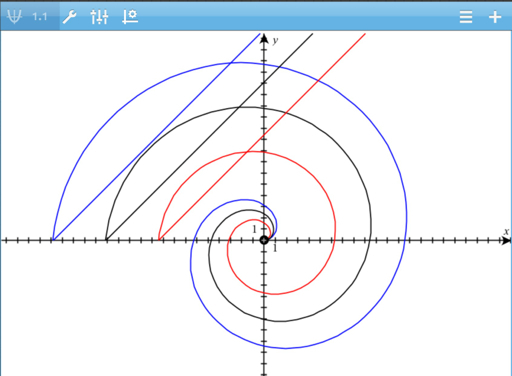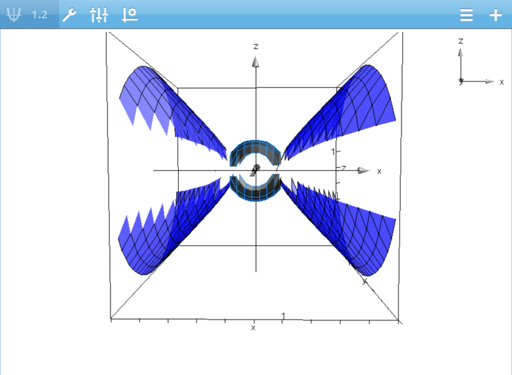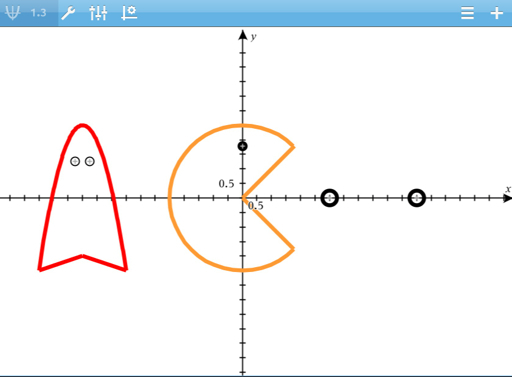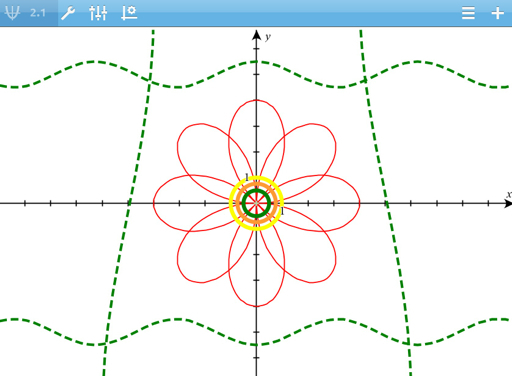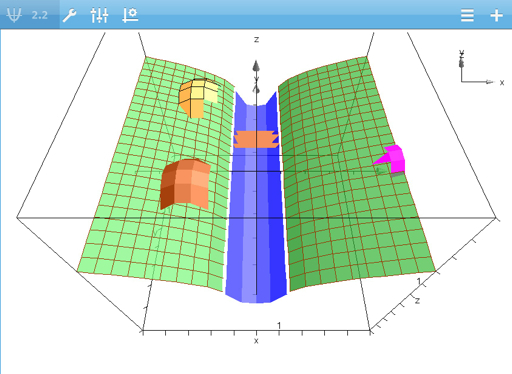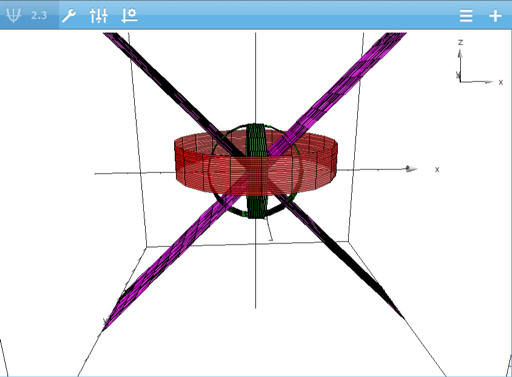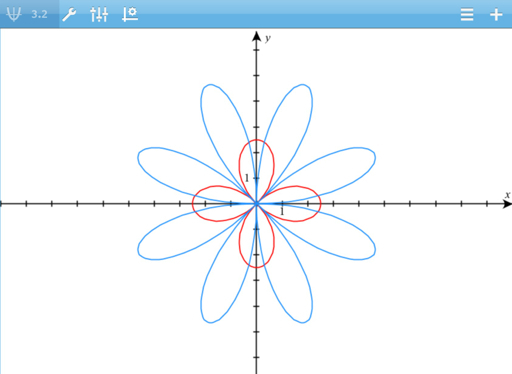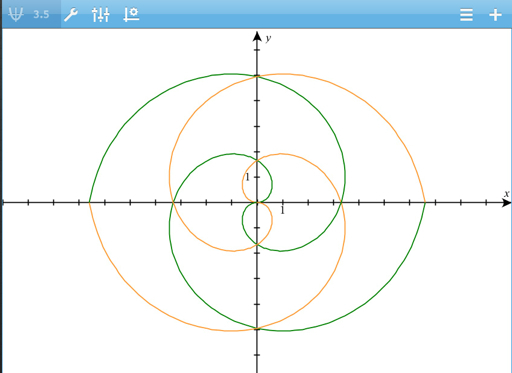## Saturday, March 9, 2013

### HP 39gii Programming Part 2: IF/THEN/ELSE/END, MSGBOX, CHOOSE

1. Comparative Operators
2. Storing and assignments
3. IF-THEN-ELSE-END
4. MSGBOX
5. CHOOSE

1. Comparative Operators

The six comparative operators are:

== Equals (Two equal signs)
≠ Not Equals
> Greater Than
≥ Greater or Equal Than
< Less Than
≤ Less or Equal Than

The result of each comparison is either 1 (the comparison is true) or 0 (the comparison is false).

Keystrokes: [Math] [X,T,θ,N],
[ 1 ] for <
[ 3 ] for ==
[ 4 ] for >
[ 9 ] for ≠
[Vars] for ≤
[Math] for ≥

2. Storing and Assignment

There are two ways to store objects:

One is by the STO> command, which usually lives in [ F1 ].

Example: 11>A

The other is by the construct := (colon then equals sign). Keystrokes [ALPHA] [ . ] [SHIFT] [ . ]

Example: A:=11

I am not sure there is any difference between the two storing methods. In this tutorial I will use the latter. (colon followed by an equals sign)

3. IF-THEN-ELSE-END

The structure of IF-THEN-ELSE-END:
IF comparison
THEN commands if the comparison is true;
ELSE commands if the comparison is false;
END;

The ELSE part is optional.

Let's use the IF-THEN-ELSE-END structure in an example. The program ISEVEN, which uses a pass-through argument, tests whether the given number is odd or even.

I usually type the IF-THEN-ELSE-END structure. In the programming edit mode, you can also insert the template with the following keystrokes: [ F6 ] (TEMPLT) [ 2 ] [ 2 ].

The program ISEVEN
EXPORT ISEVEN(N)
BEGIN
N:=INT(N);
IF FRAC(N/2)==0
THEN PRINT(string(N) + " is even.");
ELSE PRINT(string(N) + " is odd.);
END;

Testing:

ISEVEN(25) prints "25 is odd."
ISEVEN(66) prints "66 is even."

4. MSGBOX

MSGBOX is a nice way to display messages in pop-up dialog boxes.

Syntax:
MSGBOX(string of text);

Either type the command or use the following keystroke sequence: [SHIFT] [Math] [ F1 ] [ 5 ] [ 8 ]

Let's demonstrate the use of MSGBOX in the program KEPLER3. KEPLER3 demonstrates Kepler's Third Law. This program in particular tells you how many days an object orbits the Sun of our Solar System. Using U.S. units (I will list SI units too, but the program I demonstrate will use U.S. units)

The mass of the sun is approximately 4.38521 x 10^30 lbs. (1.9891 x 10^30 kg)

The Universal Gravitational constant is about 1.069104 x 10^-9 ft^3/(lb s^2).
(6.67428 x 10^-11 m^3/(kg s^2))

There are 86,400 seconds in a day.

KEPLER3 will also demonstrate the use of local variables. I recommend typing the command LOCAL. This program uses a pass-through argument, average radius.

The Program KEPLER3
EXPORT KEPLER3(R)
BEGIN
LOCAL G,M,T;
G:=1.069104E-9;
M:=4.38521E30;
T:=√((4*π^2*R^3)/(G*M))/86400;
MSGBOX("Time in Days:" + string(T));
END;

Access the "E" in numbers (stands for 10^) by pressing [SHIFT] [X,T,θ,N] (EEX).

Test: If the Earth orbits the Sun in a (near) circular motion maintaining a radius of approximately 490,806,662,402 feet, then approximately how many days does it take the Earth to orbit the sun?

KEPLER3(490806662402) returns in a pop-up box, "Time in Days:365.19645656".

Reasonable estimate don't you think?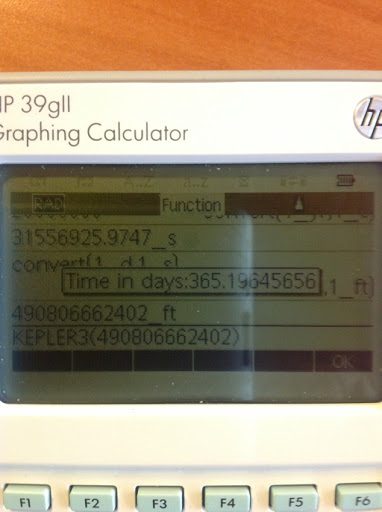5. CHOOSE

The syntax of the CHOOSE command is:
CHOOSE(var, "Title string", "Choice1", "Choice2", ...);

The choice number is stored in var.

CHOOSE can be typed or called using the keystroke sequence [SHIFT] [Math] [ F1 ] [ 5 ] [ 1 ].

If the user cancels out of the choose box, then var will have the value of 0. var can either be a global real variable (A-Z, θ) or a local variable.

The program AREAS will offer the use a choice to calculate the area of three objects: a circle, a ring, or an ellipse. The area of the circle is attached to choice 1, the area of a ring is attached to choice 2, and the area of the ellipse is attached to choice 3.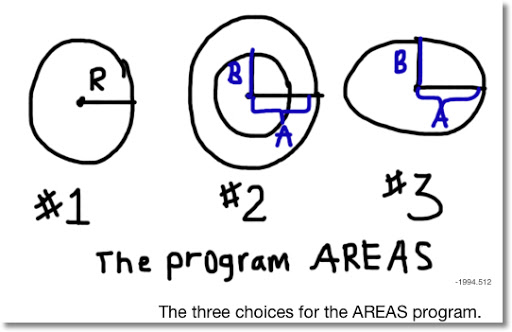Caution: Do NOT use CASE. Despite the fact that the command exists in the calculator, the programming language does not support this command. I honestly have no idea whether the next update will correct this.

The program AREAS
EXPORT AREAS()
BEGIN
CHOOSE(C,"Shape","Circle","Ring","Ellipse");
IF C==1 THEN
MSGBOX("Area="+string(π*R²));
END;
IF C==2 THEN
R:=π*(B^2-A^2);
MSGBOX("Area="+string(R));
END;
IF C==3 THEN
INPUT(A,"Semi-Axis 1");
INPUT(B,"Semi-Axis 2");
MSGBOX("Area="+string(π*A*B));
END;
END;

Test data:

Run AREAS thrice:

A circle with radius of 7.26 should return an area of 156.585808948.

A ring with the large radius of 2.07 and small radius of 1.83 will net you an area of 2.94053072376.

An ellipse with semi-axis measurements of 2.3 and 2.6 has an area of 18.7867240685.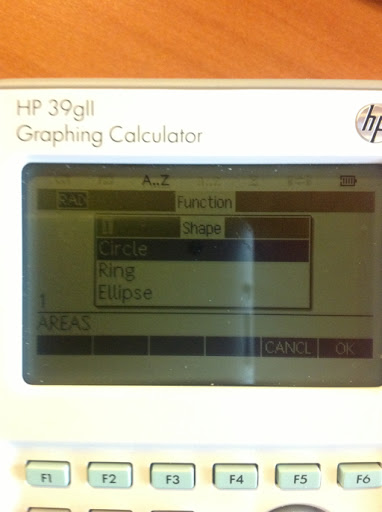In Part 3, the FOR loop will be addressed.

Until next time, Eddie

This blog is property of Edward Shore. 2013

### HP 39gii Programming Part 1: INPUT, PRINT, string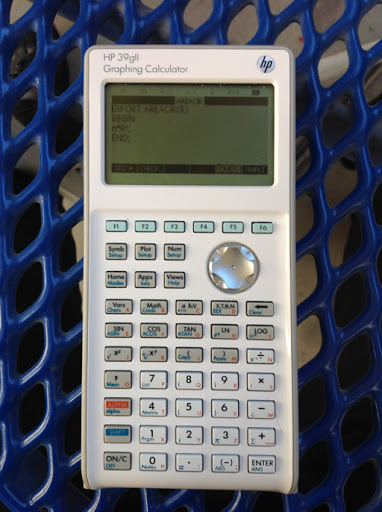Welcome to our HP 39gii Programming Boot Camp!

This is a six part tutorial series in order to get you started programming with the Hewlett Packard HP 39gii calculator. The language may be quirky and imperfect, but rest assured you can still accomplish many things with this programming language.

You can download the emulator here: http://www.hp.com/sbso/product/calculators-emulators/graphic-calculator-emulators.html. It works with Windows, it should work with the Mac (please don't quote me on this).

Contents
1. Creating a Program
2. Structure of a Program
3. The Program AREACIR
4. Running Programs
5. Running AREACIR
6. PRINT, INPUT, and string

Let's get started with our first program, AREACIR. If you guessed that this calculated the area of a circle, you are correct. Gold star for you!

1. Creating a Program
1. Press [SHIFT] [ 1 ] (PRGM).
2. Press [ F2 ] (NEW).
3. Enter the name of your new program. Use [ALPHA] for a single letter, [ALPHA] [ALPHA] to force alphabetical lock, and [SHIFT] to type lower case letters.
4. Press either [ENTER] or [ F6 ] (OK). You are off and running!

2. Structure of a Program
The general structure of a program is:

EXPORT NAME(pass-through arguments)
BEGIN
Commands;
END;

A semicolon (;) follows the end of MOST program lines, all storing commands, and mathematical calculations.

Any arguments that are passed through the program name are designated local variables. Local variables are variables that are used in the program and deleted when done. Global variables are variables that can be accessed inside and outside programming. The HP 39gii restricts the types of global variables.

Type of global variables:
Real: A through Z, θ
Matrices: M0 through M9
Lists: L0 through L9
Complex Numbers: Z0 through Z9

Almost everything else, including strings, must be local variables. To declare any variable, including global variable types, type:

LOCAL var, var, var, ...;

Include as many variables as you want. When you select LOCAL from the Cmds menu, it will give you a set parenthesis. Erase them, LOCAL does not work with parenthesis.

Let's get to our first program, AREACIR. AREACIR will have one pass-through argument, the radius.

3. The Program AREACIR
EXPORT AREACIR(R)
BEGIN
π*R²;
END;

This program returns the area of a circle to the home screen.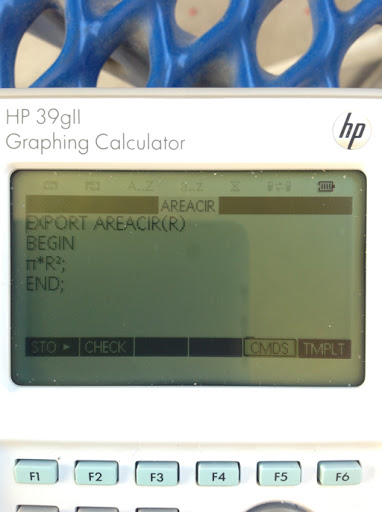4. Running Programs

You can run programs from two places: the Home Screen or thought the Program Catalog. I recommend the former (Home Screen) if you have pass-through arguments.

From the Home Screen:

1. Press [Home]
2. Press [SHIFT] [Math] (Cmds)
3. Press [ F3 ] (USER)
4. Find the program you want to run, pressing [ENTER] or [ F6 ], twice.
5. If any pass-through arguments are required, include the parenthesis and arguments. Otherwise, just press [ENTER].

You may also type out the program's with any required arguments.

5. Running AREACIR

Find the area of the circle with radius 17.5 units.

Using the Cmds menu from Home:

1. Press [Home]
2. Press [SHIFT] [Math] (Cmds)
3. Press [ F3 ] (USER)
4. Find the program you want to run, pressing [ENTER] or [ F6 ], twice.
5. Press [ ( ] 17.5 [ ) ] [ENTER]

Alternatively, you can type AREACIR(17.5) [ENTER]

The area is approximately 962.112750162.

6. PRINT, INPUT, and string

Our next program will do the same as AREACIR, except instead of using pass-through arguments, we will use prompting and displaying answers on an output terminal.

INPUT

Keystrokes: [SHIFT] [Math] [ F1 ] [ 5 ] [ 6 ]
Command can be typed
Basic Syntax: INPUT(global variable, "title string");

INPUT can have more arguments, check the HP 39gii manual. INPUT only works with one variable at a time and works only variables that can be global. INPUT does not work when promoting for strings.

In practice, I recommend that you use pass-through arguments instead of INPUT.

PRINT

Keystrokes: [SHIFT] [Math] [ F1 ] [ 5 ] [ 9 ]
Command can be typed
Basic Syntax: PRINT(string of text);

Note that strings of text are connected with the + operator.

string

This commands evaluates a mathematical expression and makes the result a string. This is useful for displaying text with mathematical calculations.

Keystrokes: [SHIFT] [Math] [ F1 ] [ 8 ] [Vars]
Command can be typed, but the command must be typed in lowercase!
Basic Syntax: string(mathematical expression or result);

Let's use INPUT, PRINT, and string in a program:

The Program AREA1:

EXPORT AREA1()
BEGIN
PRINT("THE AREA IS " + string(π*R²) + ".");
END;

Running AREA1: Type or call AREA1 at the Home Screen. Since there are no pass-through arguments, you won't need parenthesis.

You will be prompted for R. If I enter 17.5 at the prompt, I will get a screen that contains the message:

THE AREA IS 962.112750162.

When you press ENTER, that string will be returned to the Home screen.

Part 2: IF-THEN-ELSE, MSGBOX, and CHOOSE.

Have a great day!

Eddie

This blog is property of Edward Shore. 2013

## Thursday, March 7, 2013

### Update: The HP 39gii Tutorial Will Go On and Pi Day

Thanks to HP - I was able to get 39gii up and running again. I am currently writing Part 2. I aim to get at least Parts 1 and 2 up by next week.

Pi Day (March 14) is one week away! Any suggestions? How do you celebrate Pi Day?

π = 3.1415926535...

Eddie

## Tuesday, March 5, 2013

### HP 39gii Programming Series Delayed (Maybe Cancelled)

With regrets, I am going to have to delay and/or cancel the HP 39gii calculator programming tutorial series. My 39gii is giving me problems - it has an issue with freezing.

Apologies. I will give an update as soon as I can.

Eddie

This blog is property of Edward Shore. 2013

### HP 15C: Error Function and Lower Tail Normal Cumulative Function

HP 15C:   Error Function and Lower Tail Normal Cumulative Function Formulas Used  Error Function  erf(x) = 2 ÷ √π * ∫( e^(-t^2) dt, t = 0 t...